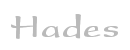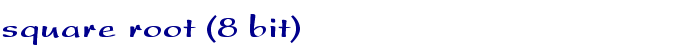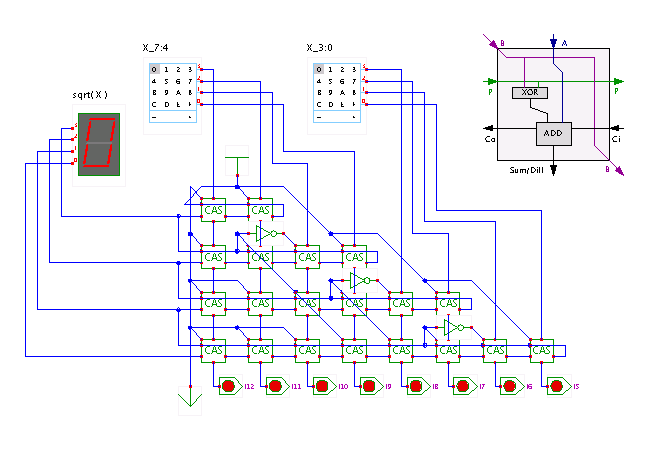TAMS / Java / Hades / applets (print version): contents | previous | next

#### Square root calculator (8 bit)Description

This circuit computes the 4-bit integer square root of the 8-bit integer input value. A four bit result is sufficient, because sqrt(255) = 15.96, which is truncated to 15.

The CAS blocks (short for controllable adder/subtractor) work as follows. If P=0, the CAS calculates (A + B + C_in), while for P=1, it calculates ((B + C_in) - A).

Run the applet | Run the editor (via Webstart)

Impressum | 24.11.06